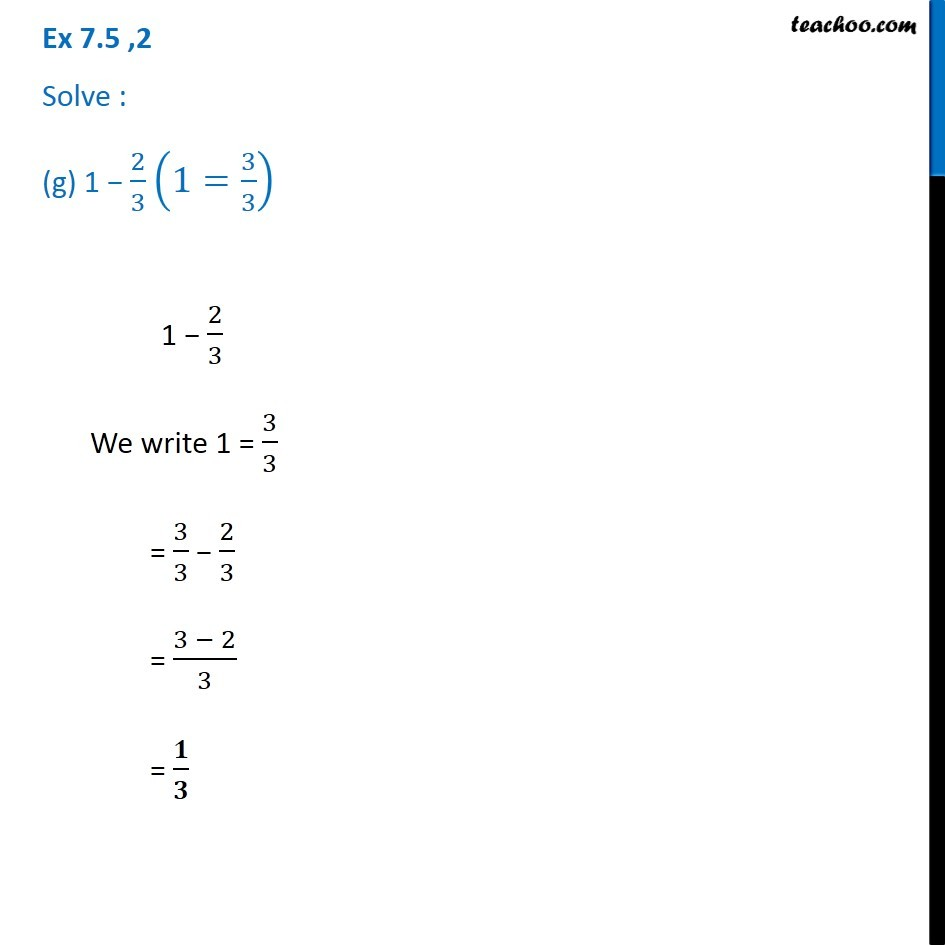1. Chapter 7 Class 6 Fractions
2. Serial order wise
3. Ex 7.5

Transcript

Ex 7.5 ,2 Solve : (g) 1 − 2/3 (1=3/3) 1 − 2/3 We write 1 = 3/3 = 3/3 − 2/3 = (3 − 2)/3 = 𝟏/𝟑